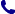• Kontakt
• Newsletter Zapisz się po informacje o nowościach i specjalnych ofertach. Administrator danych: ABE-IPS sp. z o.o.
Wybierz interesujące Cię kategorie
Wyrażam zgodę na przetwarzanie moich danych osobowych w celach marketingowych, tym samym akceptuję Regulamin Newslettera i Politykę prywatności.
Wyrażam zgodę na przesyłanie informacji handlowych drogą elektroniczną.
Wypisz się
• Klienci instytucjonalni
• Dostawa
• O nas
Dostęp on-line

# Książki

0.00 PLN
Schowek (0)
 Schowek jest pusty

## Oxford IB Diploma Programme: IB Mathematics: applications and interpretation, Higher Level, Print and Enhanced Online Course Book Pack

 Autorzy Wydawnictwo Oxford University Press Data wydania 01/03/2019 Liczba stron 832 Forma publikacji inne Poziom zaawansowania Dla szkół wyższych i kształcenia podyplomowego ISBN 9780198427049 Kategorie Matematyka
354.00 PLN (z VAT)
\$94.11 / €79.23 / £70.42 /
Produkt dostępny
Dostawa 2 dni
Ilość
Do schowka

## Opis książki

Featuring a wealth of digital content, this concept-based Print and Enhanced Online Course Book Pack has been developed in cooperation with the IB to provide the most comprehensive support for the new DP Mathematics: applications and interpretation HL syllabus, for first teaching in September 2019. Each Enhanced Online Course Book Pack is made up of one full-colour, print textbook and one online textbook - packed full of investigations, exercises, worksheets, worked solutions and answers, plus assessment preparation support.

Oxford IB Diploma Programme: IB Mathematics: applications and interpretation, Higher Level, Print and Enhanced Online Course Book Pack

## Spis treści

Measuring space: accuracy and geometry1.1: Representing numbers exactly and approximately 1.2: Angles and triangles 1.3: three-dimensional geometryRepresenting and describing data: descriptive statistics2.1: Collecting and organizing data 2.2: Statistical measures 2.3: Ways in which we can present data 2.4: Bivariate dataDividing up space: coordinate geometry, lines, Voronoi diagrams, vectors3.1: Coordinate geometry in 2 and 3 dimensions 3.2: The equation of a straight line in 2 dimensions 3.3: Voronoi diagrams 3.4: Displacement vectors 3.5: The scalar and vector product 3.6: Vector equations of linesModelling constant rates of change: linear functions and regressions4.1: Functions 4.2: Linear models 4.3: Inverse functions 4.4: Arithmetic sequences and series 4.5: Linear regressionQuantifying uncertainty: probability5.1: Theoretical and experimental probability 5.2: Representing combined probabilities with diagrams 5.3: Representing combined probabilities with diagrams and formulae 5.4: Complete, concise and consistent representationsModelling relationships with functions: power and polynomial functions6.1: Quadratic models 6.2: Quadratic modelling 6.3: Cubic functions and models 6.4: Power functions, inverse variation and modelsModelling rates of change: exponential and logarithmic functions7.1: Geometric sequences and series 7.2: Financial applications of geometric sequences and series 7.3: Exponential functions and models 7.4: Laws of exponents - laws of logarithms 7.5: Logistic modelsModelling periodic phenomena: trigonometric functions and complex numbers8.1: Measuring angles 8.2: Sinusoidal models: f(x) = asin(b(x-c))+d 8.3: Completing our number system 8.4: A geometrical interpretation of complex numbers 8.5: Using complex numbers to understand periodic modelsModelling with matrices: storing and analyzing data9.1: Introduction to matrices and matrix operations 9.2: Matrix multiplication and properties 9.3: Solving systems of equations using matrices 9.4: Transformations of the plane 9.5: Representing systems 9.6: Representing steady state systems 9.7: Eigenvalues and eigenvectorsAnalyzing rates of change: differential calculus10.1: Limits and derivatives 10.2: Differentiation: further rules and techniques 10.3: Applications and higher derivativesApproximating irregular spaces: integration and differential equations11.1: Finding approximate areas for irregular regions 11.2: Indefinite integrals and techniques of integration 11.3: Applications of integration 11.4: Differential equations 11.5: Slope fields and differential equationsModelling motion and change in 2D and 3D: vectors and differential equations12.1: Vector quantities 12.2: Motion with variable velocity 12.3: Exact solutions of coupled differential equations 12.4: Approximate solutions to coupled linear equationsRepresenting multiple outcomes: random variables and probability distributions13.1: Modelling random behaviour 13.2: Modelling the number of successes in a fixed number of trials 13.3: Modelling the number of successes in a fixed interval 13.4: Modelling measurements that are distributed randomly 13.5: Mean and variance of transformed or combined random variables 13.6: Distributions of combined random variablesTesting for validity: Spearman's hypothesis testing and x2 test for independence14.1: Spearman's rank correlation coefficient 14.2: Hypothesis testing for the binomial probability, the Poisson mean and the product moment correlation coefficient 14.3: Testing for the mean of a normal distribution 14.4: Chi-squared test for independence 14.5: Chi-squared goodness-of-fit test 14.6: Choice, validity and interpretation of testsOptimizing complex networks: graph theory15.1: Constructing graphs 15.2: Graph theory for unweighted graphs 15.3: Graph theory for weighted graphs: the minimum spanning tree 15.4: Graph theory for weighted graphs - the Chinese postman problem 15.5: Graph theory for weighted graphs - the travelling salesman problemExploration

## Polecamy również książki801 777 223